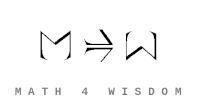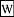文章 发现 ms@ms.lt +370 607 27 665 My work is in the Public Domain for all to share freely. 读物 书 影片 维基百科 Software Upload 数学笔记 In what sense is Faraday's pail a paradigmatic subsystem? In what sense is it the quantum harmonic oscillator? How to think of its minimal implementation and maximal implementation? In topology, is the asymmetry between arbitrary unions and finite intersections the foundation for the difference between local and global? How to solve {$x^{(x+1)}=(x+1)^x$}? Those things are which show themselves to be. Colimit (inner structure - unconscious) shows itself by limit (external relationships - conscious). In physics, everything is action. Action gets divided into perspectives, as with angular momentum: p r = m v r. Feynman diagram. Momentum k is attributed to lines. Steps in the combinatorics of orthogonal polynomials express momentum. Nature's language is limited at the smallest scales, which is the basis for quantum effects, as it must simplify to provide an answer, and can't be perfectly nuanced. Measurement and experiments take place with regard to action so quantizing action (whether in the smallest scales or largest scales) quantizes experiments and measurement. The adjunction of free construction (free will) and forgetful (fate) functors expresses the twosome. Think of action as A= Fxt where F=ma. Also, A=Et. A=px. Action is the size of a subsystem. How is action related to force? How is action related to slack? Angular momentum is a representation of action. Would it make sense to have a quantum giving the maximal size of an action, that is, a subsystem? What if there is an upper limit on action, a maximal quantum? And could such a constraint explain renomalisation? But also explain the acceleration of the expansion of the universe when a certain age t is reached, so that tE becomes too large, and so the system must be restructured, broken down by time into smaller systems? So that this restructuring yields a force? Or similarly as the size of the universe increases to a certain x, then p x must be restructured, so that the universe consists of smaller systems? Could such quantization from above explain dark energy and dark matter? Why is the matrix for M for relativistic angular momentum given as {$x^ap^b-x^bp^a$}? The units make sense. Fermionic minimal answer is "yes or no": -1/2 (no) or +1/2 (yes). Bosonic spin-0 minimal answer is "I don't know" 0. Spin-1 has three answers. Spin-2 for the graviton has five answers, as with the fivesome. Thus spin can express divisions of everything. Studyspinors and Clifford algebras. Relate to angular momentum. Adjunction gives the kinds of dualities and trivialities. Quantizing action A affects dA, the principle of least action, the conservation laws. Is there a meaningful way of thinking about the Hamiltonian K+V as symmetric and the Lagrangian K-V as antisymmetric? Those things are which show themselves to be. Shulman: By analogy with function extensionality and propositional extensionality, univalence could be called typal extensionality. In particular, like function extensionality and propositional extensionality, univalence is an “extensionality” property, meaning that “types are determined by their behavior.” 4+6 The conservation laws for 4-momentum {$p_a$} and 6-angular momentum {$M^{ab}$} arise respectively from the 4 translational symmetries and 6 (Lorentz) rotational symmetries of Minkowski space. The tensor quantity {$M^{ab}$} describes angular momentum in special relativity. This is what I need to quantize. And {$M^{ab}=x^ap^b-x^bp^a$} relates six pairs of four dimensions, including time. Compare with pairs of levels as given by the six qualities of signs. Thus "the thing" is given by time and the dimensions of space are simply signs of time of three different kinds: icon, index, symbol. https://en.m.wikipedia.org/wiki/Noether%27s_theorem based on action. Symmetry of coordinate system yields conservation law. For Thomas: Epistemology of biology How epistemology of biology relates to epistemology of physics and math. Big picture indefinite, definite, imaginable, unimaginable those things are which show themselves to be: God being somebody's God: category theory - inner structure to external relationships The kinds of oneness. The structures: 4 meanings, 4x4 equation of life, 4 flows, 4 persons. Fostering the gap between God beyond us and God within us. Derivation of 6 representations, 12 circumstances. Physics: Understanding why the 4th power in the Feynman diagrams. Physics: How does quantization work (of E or E2, etc.) and what would it mean to quantize angular momentum. Those things are which show themselves to be. This means that internal structure must get expressed through external relationships, as with category theory. Colimits are internal structures and limits are external relationships. Thus colimits need to get expressed as limits. A topos has arbitrary colimits and finite limits, just as a topology has arbitrary unions and finite intersections. Right adjoint functors preserve limits and left adjoint functors preserve colimits. Thus we should move from left adjoint functors (such as adding perspectives, as with free constructions) to right adjoint functors (removing perspectives, as with forgetting). Free construction moves us from kinetic energy to potential energy. Forgetting moves us from potential energy to kinetic energy. How would we quantize angular momentum instead of energy? It is naturally periodic. Time has direction when there is a charge so it can be reversed with opposite charge. Marked opposite? Tensor product - thinking-in-parallel - homogeneity of choice (geometry). Homset - thinking-in-series - step-by-step (algebra). Feynman diagrams. Ask Thomas: Why 4 branches and not 3 or 6 or 8 and so on? Time is no perspective, space is p, p2, p3, these are the four geometries. So time is affine. https://en.m.wikipedia.org/wiki/AdS/CFT_correspondence There are field theories in four dimensions which at strong coupling become quantum gravity theories in ten dimensions! The strong cou- pling effects cause the excitations to act as if they’re gravitons moving in higher dimensions. This is quite extraordinary and still poorly u Relate type theory to amounts and units. Math discovery. Equality is self-superposition in threesome. Thus 3 is 5-2. Then extend domain. Continuity (homotopy ?) Defines critical point. any object f : B −→ A of a slice category C /A has a universal property: it is the image of the terminal object 1B : B −→ B of C /B under the left adjoint f! : C /B −→ C /A to pullback along f . Ramybė sąlygiškas buvimas nebuvime. Quantum foam.
Šis puslapis paskutinį kartą keistas December 03, 2021, at 02:54 PM